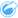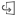# Calculate Density Ratio

Calculate Density Ratio uses two input point datasets to calculate a spatial relative risk surface. The numerator in the ratio represents cases, such as number of crimes or number of patients, and the denominator represents the control, such as the total population.

Calculate Density Ratio uses the same calculations to create density surfaces as Calculate Density. The outputs of Calculate Density and Calculate Density Ratio may appear similar; however, the output of Calculate Density Ratio is normalized, meaning it displays a proportional value, whereas the output of Calculate Density is not. Use a density ratio when the phenomenon being analyzed requires a control, such as total population.

The individual density surfaces are calculated using Kernel density before the ratio is calculated.

## Example

An epidemiologist is studying the occurrences of a disease to determine if high occurrences in certain areas could be linked to environmental factors. The density ratio is calculated using the disease occurrences as the numerator and the total population as the denominator. The result surface shows the density of disease occurrence normalized by population density, which makes it possible to determine where the disease occurrences are higher than expected.

See the Create a COVID-19 relative risk surface blog for a complete workflow.

## Use the Calculate Density Ratio capability

You can run Calculate Density Ratio on maps with point layers. Two separate datasets are required as input.

Complete the following steps to run the Calculate Density Ratio analysis capability:

1. If necessary, click the map card to activate it. A card is active when the toolbar and Action buttonappear.
2. Click the Action button and choose Calculate Density Ratio.
3. For Choose point layers, select the layers you want to use for the numerator and denominator.
4. Expand Additional options and enter values for the Weight (numerator and denominator), Search radius (bandwidth) (numerator and denominator), and Cell size parameters, if necessary.
5. Click Run.

## Usage notes

The Choose point layers parameter is used to select datasets to calculate densities for the numerator and denominator. Only point features are available as inputs.

Expand Additional options to reveal the Weight, Search radius (bandwidth), and Cell size parameters. The following table summarizes these parameters, including their default values:

ParameterDescriptionDefault value

Weight

A field denoting the value of each feature. For example, if you have a dataset for retail locations that includes a field for revenue, you could use the revenue field as the weight to create a density surface based on sales amount rather than locations.

The Weight fields are applied to the numerator and denominator separately.

All features are weighted as 1 (in other words, the density surface is based solely on the location of the features).

A distance (in miles, feet, kilometers, or meters) that is used to find input features within the same neighborhood as the focal feature.

The Search radius (bandwidth) fields are applied to the numerator and denominator separately.

An appropriate search distance is calculated for the input dataset using Silverman's Rule-of-thumb formula.

The search distance is calculated separately for the numerator and denominator.

Cell size

The size of the output features that will create the density ratio surface.

An appropriate cell size is calculated based on the extent and number of features in the input datasets.

The result dataset is styled by Counts and amounts (Color) using a default equal interval classification with 10 classes.

The Flip card buttonis used to view information on the back of the card, including the values for the search radius (numerator and denominator) and bandwidth.

## Limitations

The input dataset must be point features.

Cross filters are not compatible with result datasets created by this tool. If you want to add a cross filter to an unsupported card, you can copy the dataset to your workbook and apply a cross filter to the cards created with the copy.

This tool is not supported for read-only connections to Google BigQuery, Snowflake, and database platforms that are not supported out-of-the-box.

## How Calculate Density Ratio works

Calculate Density Ratio uses Kernel density calculations to create density surfaces; it then calculates a ratio between each surface.

The ratio is calculated between the density surfaces using the following equation:

``r(x, y)= c(x, y)/p(x, y)``

Where:

• r(x,y) = Ratio at location (x,y).
• c(x,y) = Density of cases (numerator) at location (x,y).
• p(x,y) = Density of control (denominator) at location (x,y).

For more information about Kernel density calculations, including the default calculations for Search radius (bandwidth) and Cell size, see How Calculate Density works.